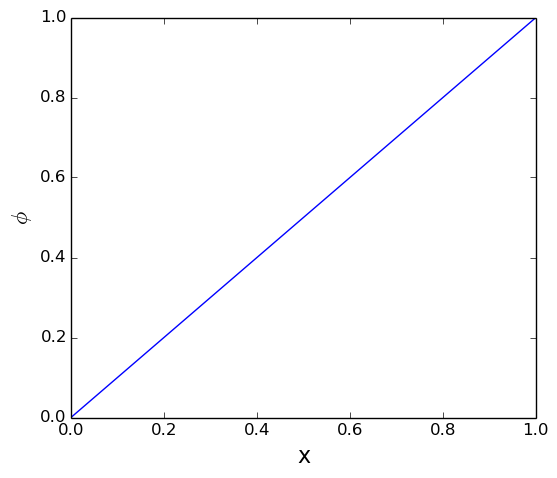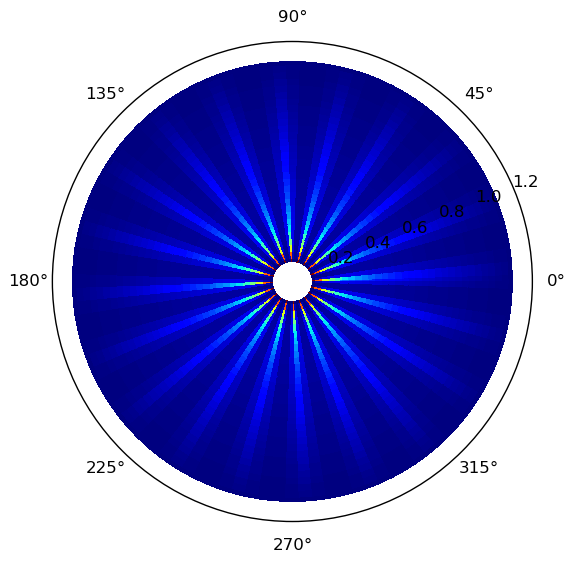# JFVM: a finite volume tool for Julia

During the Christmass holydays, I spent some time to convert my Matlab FVTool to a Julia package. The result, which I call JFVM is a bit Matlabesque, but the package works. In this post I'm going to show how it works.

### Installation¶

To install the package, you can use Pkg.add("JFVM") in Julia, or clone the most recent version (recommended). You need to have Julia installed. JFVM relies on PyPlot, PyCall, and Mayavi for visualization. Here's the procedure.

#### Linux¶

You need to first install Python, Matplotlib, and Mayavi. To do it on an Ubuntu-based system, try

sudo apt-get install python2.7 python-matplotlib mayavi2

Then go to your .julia/v0.4 or .julia/v0.3 (recommended) folder and type

git clone https://github.com/simulkade/JFVM.jl.git

#### Windows¶

There used to be a few issues with 3D visualization in windows. The current version however should work fine. This is the workflow if you want to give it a try:

• Run anaconda command prompt (as administrator) and install mayavi and wxpython:
• conda install mayavi
• conda install wxpython (Not necessary if you clone the last version of JFVM)
• Install github for windows
• open github shell, go to .julia/v0.4 or .julia/v0.3 and type:
• git clone https://github.com/simulkade/JFVM.git

Please let me know if it does not work on your windows machines.

### What does it do?¶

It solves a transient convection-diffusion equation, i.e., $$\alpha\frac{\partial\phi}{\partial t}+\nabla.\left(\mathbf{u}\phi\right)+\nabla.\left(-D\nabla\phi\right)+\beta\phi=\gamma$$ with the boundary condition: $$a\nabla\phi.\mathbf{n}+b\phi=c.$$ Each term, i.e., transient, convection, diffusion, linear source, and constant source terms can be called via a separate function, which makes it possible to use the code for solving various coupled and even non-linear convection-diffusion equations. The main difference between this code and the previous Matlab version is that in this one, nonuniform grids can be defined. The supported coordinates are

• 1D axisymmetric (radial)
• 2D radial (r, theta)
• 2D Cartesian
• 3D Cartesian
• 2D axisymmetric (cylindrical, r, z)
• 3D cylindrical (r, theta, z) One other thing that I always had in mind is the possibility to use hetrogeneous transfer coefficients, e.g., a diffusion coefficient that is different on each cell. In addition, I wanted to be able to define different boundary conditions on the faces of the boundary cell. The last feature that I constantly miss in other PDE solvers is the Robin boundary condition, which can be defined quite easily in JFVM.
One last feature that I added in later stages was the periodic boundary condition.

### A simple example¶

This is perhaps the simplest example: a diffusion equation on a 1D domain, with a Dirichlet boundary on each side. The equation read $$\nabla (-D \nabla \phi) = 0$$ where $\phi$ is zero on the left and one on the right side of the domain. The formulation in JFVM always follows this sequence:

• Create a domain and mesh
• Create a boundary for the domain (default: Neumann)
• Change the boundary condition (optional)
• Define the initial condition (optional, only if you have transient terms)
• Define the transfer coefficients
• Calculate the matrix of coefficients and RHS vector for each transfer term in the PDE
• Call the linear solver
• Call the visualization tool There can be other steps in between depending on the problems.
In :
using JFVM, PyPlot

In :
L= 1.0 # length of the domain
Nx= 20 # number of cells
m= createMesh1D(Nx, L) # create the domain and mesh
BC= createBC(m) # create the boundary condition
BC.left.a[:]= 0.0 # left side Neumann term equal to zero
BC.left.b[:]= 1.0 # left side Dirichlet term coefficient equal to one
BC.left.c[:]= 0.0 # left side Dirichlet term value equal to zero
BC.right.a[:]= 0.0
BC.right.b[:]= 1.0
BC.right.c[:]= 1.0
D_val= 1.0 # value of the transfer coefficient
D= createCellVariable(m, D_val) # assign D_val to all the cells
# harmonic average of the transfer coefficient on the cell faces:
D_face= harmonicMean(D)
(Mbc, RHSbc)= boundaryConditionTerm(BC)
Mdiff= diffusionTerm(D_face)
M= Mdiff+Mbc # matrix of coefficients
RHS= RHSbc # off course!
phi= solveLinearPDE(m, M, RHS) # solve the linear PDE
figure(figsize=(6.0,5.0)) # unit is [cm] for me
visualizeCells(phi) # visualize the results
# decorate the plot
xlabel("x", fontsize=16)
ylabel(L"\phi", fontsize=16)Out:
PyObject <matplotlib.text.Text object at 0x7fa9bb423310>

### A colorful a bit playful case¶

The other night, my daughter wanted to see what I do with my computer. So I decided to solve an equation with fancy colorful results. So I created a diffusion process on a radial coordinate, Dirichlet boundary condition near the center of the circle (with alternationg zero-one values on the left boundary). I reduced the value of the diffusion coefficient in the direction of increasing $\theta$ to increase the contrast in the result. Let me recreate it here.

In :
L= 1.0 # length of the domain
Nx= 30 # number of cells
Ntheta= 5*20
m= createMeshRadial2D(Nx, Ntheta, L, 2π) # create the domain and mesh
m.cellcenters.x+=0.1
m.facecenters.x+=0.1
BC= createBC(m) # create the boundary condition
BC.left.a[:]= 0.0 # left side Neumann term equal to zero
BC.left.b[:]= 1.0 # left side Dirichlet term coefficient equal to one
BC.left.c[:]= 0.0 # left side Dirichlet term value equal to zero
BC.left.c[1:5:Ntheta]= 1.0
BC.right.a[:]= 0.0
BC.right.b[:]= 1.0
BC.right.c[:]= 0.0
BC.bottom.periodic=true # periodic boundary condition
D_val= 1.0 # value of the transfer coefficient
D= createCellVariable(m, D_val) # assign D_val to all the cells
# harmonic average of the transfer coefficient on the cell faces:
D_face= harmonicMean(D)
D_face.yvalue[:]=0.003*D_val
(Mbc, RHSbc)= boundaryConditionTerm(BC)
Mdiff= diffusionTerm(D_face)
M= -Mdiff+Mbc # matrix of coefficients
RHS= RHSbc # off course!
phi= solveLinearPDE(m, M, RHS) # solve the linear PDE
visualizeCells(phi) # visualize the resultsOut:
PyObject <matplotlib.collections.PolyCollection object at 0x7fa9b9744350>

### Not really bad!¶

That's it for now. I will come back with more real life examples.